Next: The linear approximation Up: THE LARGE-SCALE STRUCTURE OF Previous: The standard cosmological model

# Describing the inhomogeneities of the Universe

Cosmic inhomogeneities are usually described by the fluctuation field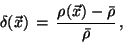(7)

where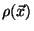is the density of the Universe at the point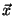. A popular statistical characterization of the inhomogeneities in the distribution of cosmic structures is provided by the two-point correlation function,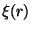, which describes the expected excess fluctuations with respect to a uniform distribution: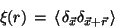(8)

where the symbol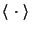indicates the average over all the pairs of points at separation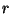. Its Fourier transform is the power spectrum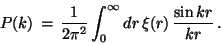(9)

If clustering is isotropic, thenand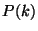depend only on the modulus of the separation- and wave-vectors, respectively. The power spectrum of the observed galaxy and cluster distributions contains formally the same amount of information as, although the practical computation of the two quantities is differently affected by the systematics of redshift surveys.

Another important statistical characterisation is provided by the variance,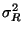, of the mass density fluctuations within a sphere of radius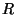: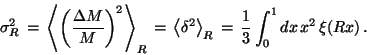(10)

Galaxy surveys show that for normal galaxies the variance in the galaxy number counts is about unity for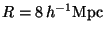[7,21]. For this reason, it is customary to specify the normalisation of the power spectrum at this scale, so that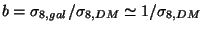provides the so-called galaxy biasing with respect to the dark matter distribution. Therefore, by definition, the galaxy biasing provides a measure of the difference between the distribution of the observable light and the distribution of the unseen cosmic dark matter.

Observations of the large-scale distribution of cosmic structures shows that the two-point correlation function for both galaxies and galaxy clusters can be well approximated by a power law,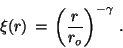(11)

Although the slope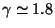is quite simila i.e. the correspondence between background and physical space-time) in which fluctuation measurements are performed. In this case, a proper gauge-invariant description of fluctuation evolution is required and several prescriptions have been proposed by different authors (see ref. for a recent review on this subject). At the present time, all the scales relevant for clustering studies are are well inside the horizon. However, since perturbation wavelengths scale with the redshift as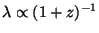and the horizon as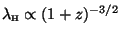, the typical scale of galaxy clustering,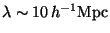, crosses the horizon at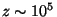, roughly corresponding to the epoch of matter-radiation equality. Thus, a gauge invariant treatment is required when studying the formation of density fluctuations relevant to the present observed clustering. Here we adopt a purely Newtonian treatment, which is adequate to follow the perturbation evolution after recombination, when structure formation starts.

Passing to the Fourier representation of the density fluctuation field, the system () becomes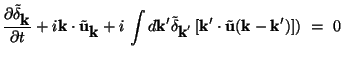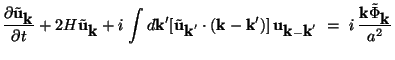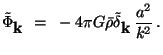(12)

Eqs. (12) and () can be equivalently used as the non-linear systems describing the evolution of cosmic density fluctuations. In the following we will describe some approximations that have been proposed in order to get hints about the nature of gravitational clustering. In the next Chapter we will discuss the use of cosmological N-body simulations as a tool to follow in detail the non-linear dynamics implied by eqs.(12).

SubsectionsNext: The linear approximation Up: THE LARGE-SCALE STRUCTURE OF Previous: The standard cosmological model
Waleska Aldana Segura 2001-01-16